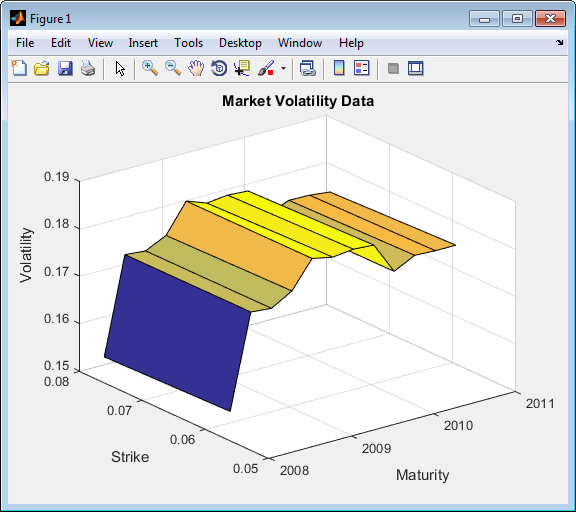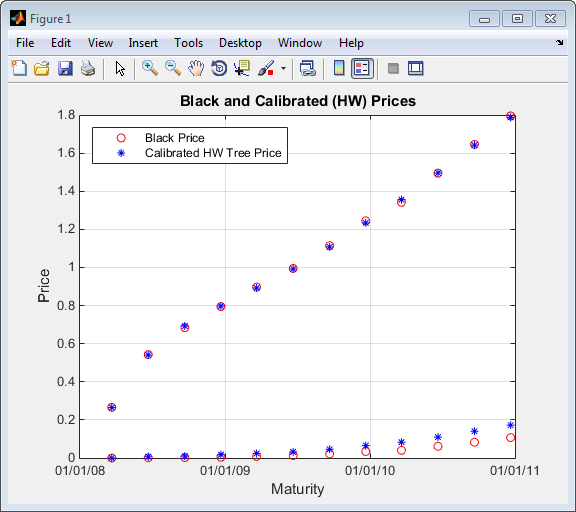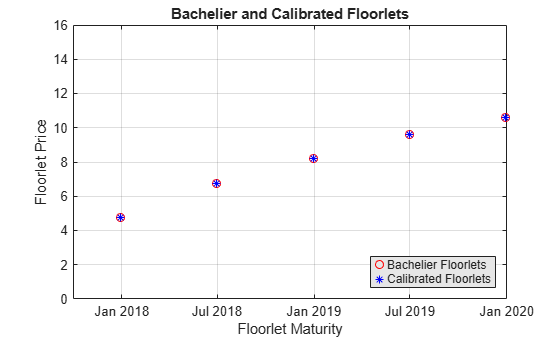# hwcalbyfloor

Calibrate Hull-White tree using floors

## Syntax

``[Alpha,Sigma,OptimOut] = hwcalbyfloor(RateSpec,MarketStrikeMarketMaturity,MarketVolatility)``
``[Alpha,Sigma,OptimOut = hwcalbyfloor(RateSpec,MarketStrikeMarketMaturity,MarketVolatility,Strike,Settle,Maturity)``
``[Alpha,Sigma,OptimOut] = hwcalbyfloor(___,Name,Value)``

## Description

example

````[Alpha,Sigma,OptimOut] = hwcalbyfloor(RateSpec,MarketStrikeMarketMaturity,MarketVolatility)` calibrates the `Alpha` (mean reversion) and `Sigma` (volatility) using floor market data and the Hull-White model using the entire floor surface. The Hull-White calibration functions (`hwcalbyfloor` and `hwcalbycap`) support three models: Black (default), Bachelier or Normal, and Shifted Black. For more information, see the optional arguments for `Shift` and `Model`.```

example

````[Alpha,Sigma,OptimOut = hwcalbyfloor(RateSpec,MarketStrikeMarketMaturity,MarketVolatility,Strike,Settle,Maturity)` estimates the `Alpha` (mean reversion) and `Sigma` (volatility) using floor market data and the Hull-White model to price a floor at a particular maturity/volatility using the additional optional input arguments for `Strike`, `Settle`, and `Maturity`. `Strike`, `Settle`, and `Maturity` arguments are specified to calibrate to a specific point on the market volatility surface. If omitted, the calibration is performed across all the market instrumentsFor an example of calibrating using the Hull-White model with `Strike`, `Settle`, and `Maturity` input arguments, see Calibrating Hull-White Model Using Market Data.```

example

````[Alpha,Sigma,OptimOut] = hwcalbyfloor(___,Name,Value)` adds optional name-value pair arguments. ```

## Examples

collapse all

This example shows how to use `hwcalbyfloor` input arguments for `MarketStrike`, `MarketMaturity`, and `MarketVolatility` to calibrate the HW model using the entire floor volatility surface.

Floor market volatility data covering two strikes over 12 maturity dates.

```Reset = 4; MarketStrike = [0.0590; 0.0790]; MarketMaturity = {'21-Mar-2008'; '21-Jun-2008'; '21-Sep-2008'; '21-Dec-2008'; '21-Mar-2009'; '21-Jun-2009'; '21-Sep-2009'; '21-Dec-2009'; '21-Mar-2010'; '21-Jun-2010'; '21-Sep-2010'; '21-Dec-2010'}; MarketMaturity = datenum(MarketMaturity); MarketVolaltility = [0.1533 0.1731 0.1727 0.1752 0.1809 0.1800 0.1805 0.1802... 0.1735 0.1757 0.1755 0.1755; 0.1526 0.1730 0.1726 0.1747 0.1808 0.1792 0.1797 0.1794... 0.1733 0.1751 0.1750 0.1745]; ```

Plot market volatility surface.

```[AllMaturities,AllStrikes] = meshgrid(MarketMaturity,MarketStrike); figure; surf(AllMaturities,AllStrikes,MarketVolaltility) datetick xlabel('Maturity') ylabel('Strike') zlabel('Volatility') title('Market Volatility Data') ```Set interest rate term structure and create a `RateSpec`.

```Settle = '21-Jan-2008'; Compounding = 4; Basis = 0; Rates= [0.0627; 0.0657; 0.0691; 0.0717; 0.0739; 0.0755; 0.0765; 0.0772; 0.0779; 0.0783; 0.0786; 0.0789]; EndDates = {'21-Mar-2008';'21-Jun-2008';'21-Sep-2008';'21-Dec-2008';... '21-Mar-2009';'21-Jun-2009';'21-Sep-2009';'21-Dec-2009';.... '21-Mar-2010';'21-Jun-2010';'21-Sep-2010';'21-Dec-2010'}; RateSpec = intenvset('ValuationDate', Settle, 'StartDates', Settle,... 'EndDates', EndDates,'Rates', Rates, 'Compounding', Compounding,... 'Basis',Basis) ```
```RateSpec = FinObj: 'RateSpec' Compounding: 4 Disc: [12x1 double] Rates: [12x1 double] EndTimes: [12x1 double] StartTimes: [12x1 double] EndDates: [12x1 double] StartDates: 733428 ValuationDate: 733428 Basis: 0 EndMonthRule: 1```

Calibrate Hull-White model from market data.

```o = optimoptions('lsqnonlin','TolFun',1e-5,'Display','off'); [Alpha, Sigma] = hwcalbyfloor(RateSpec, MarketStrike, MarketMaturity,... MarketVolaltility, 'Reset', Reset,'Basis', Basis, 'OptimOptions', o) ```
```Warning: LSQNONLIN did not converge to an optimal solution. It exited with exitflag = 3. > In hwcalbycapfloor>optimizeOverCapSurface at 232 In hwcalbycapfloor at 79 In hwcalbyfloor at 81 Alpha = 0.0835 Sigma = 0.0145 ```

Compare with Black prices.

```BlkPrices = floorbyblk(RateSpec,AllStrikes(:), Settle, AllMaturities(:),... MarketVolaltility(:),'Reset',Reset,'Basis',Basis)```
```BlkPrices = 0 0.2659 0.0010 0.5426 0.0021 0.6841 0.0042 0.7947 0.0081 0.8970 0.0128 0.9947 0.0217 1.1145 0.0340 1.2448 0.0402 1.3415 0.0610 1.4947 0.0827 1.6458 0.1071 1.7951 ```

Setup Hull-White tree using calibrated parameters, alpha, and sigma.

```VolDates = EndDates; VolCurve = Sigma*ones(numel(EndDates),1); AlphaDates = EndDates; AlphaCurve = Alpha*ones(numel(EndDates),1); HWVolSpec = hwvolspec(Settle, VolDates, VolCurve, AlphaDates, AlphaCurve); HWTimeSpec = hwtimespec(Settle, EndDates, Compounding); HWTree = hwtree(HWVolSpec, RateSpec, HWTimeSpec, 'Method', 'HW2000') ```
```HWTree = FinObj: 'HWFwdTree' VolSpec: [1x1 struct] TimeSpec: [1x1 struct] RateSpec: [1x1 struct] tObs: [0 0.6593 1.6612 2.6593 3.6612 4.6593 5.6612 6.6593 7.6612 8.6593 9.6612 10.6593] dObs: [733428 733488 733580 733672 733763 733853 733945 734037 734128 734218 734310 734402] CFlowT: {1x12 cell} Probs: {1x11 cell} Connect: {1x11 cell} FwdTree: {1x12 cell}```

Compute Hull-White prices based on the calibrated tree.

`HWPrices = floorbyhw(HWTree, AllStrikes(:), Settle, AllMaturities(:), Reset, Basis) `
```HWPrices = 0 0.2644 0.0067 0.5404 0.0101 0.6924 0.0169 0.7974 0.0236 0.8919 0.0320 0.9919 0.0460 1.1074 0.0649 1.2340 0.0829 1.3558 0.1096 1.4957 0.1406 1.6418 0.1724 1.7877```

Plot Black prices against the calibrated Hull-White tree prices.

```figure; plot(AllMaturities(:), BlkPrices, 'or', AllMaturities(:), HWPrices, '*b'); datetick('x', 2) xlabel('Maturity'); ylabel('Price'); title('Black and Calibrated (HW) Prices'); legend('Black Price', 'Calibrated HW Tree Price','Location', 'NorthWest'); grid on ```This example shows how to use `hwcalbyfloor` to calibrate market data with the Normal (Bachelier) model to price floorlets. Use the Normal (Bachelier) model to perform calibrations when working with negative interest rates, strikes, and normal implied volatilities.

Consider a floor with these parameters:

```Settle = datetime(2016,12,30); Maturity = datetime(2019,12,30); Strike = -0.004075; Reset = 2; Principal = 100; Basis = 0;```

The floorlets and market data for this example are defined as:

```floorletDates = cfdates(Settle, Maturity, Reset, Basis); datestr(floorletDates')```
```ans = 6x11 char array '30-Jun-2017' '30-Dec-2017' '30-Jun-2018' '30-Dec-2018' '30-Jun-2019' '30-Dec-2019' ```
```% Market data information MarketStrike = [-0.00595; 0]; MarketMat = [datetime(2017,6,30) ; datetime(2017,12,30) ; datetime(2018,6,30) ; datetime(2018,12,30) ; datetime(2019,6,30) ; datetime(2019,12,30)]; MarketVol = [0.184 0.2329 0.2398 0.2467 0.2906 0.3348; % First row in table corresponding to Strike 1 0.217 0.2707 0.2760 0.2814 0.3160 0.3508]; % Second row in table corresponding to Strike 2```

Define the `RateSpec` using `intenvset`.

```Rates= [-0.003210;-0.003020;-0.00182;-0.001343;-0.001075]; ValuationDate = datetime(2016,12,30); EndDates = [datetime(2017,6,30) ; datetime(2017,12,30) ; datetime(2018,6,30) ; datetime(2018,12,30) ; datetime(2019,12,30)]; Compounding = 2; Basis = 0; RateSpec = intenvset('ValuationDate', ValuationDate, ... 'StartDates', ValuationDate, 'EndDates', EndDates, ... 'Rates', Rates, 'Compounding', Compounding, 'Basis', Basis);```

Use `hwcalbyfloor` to find values for the volatility parameters `Alpha` and `Sigma` using the Normal (Bachelier) model.

```format short o=optimoptions('lsqnonlin','TolFun',100*eps); warning ('off','fininst:hwcalbycapfloor:NoConverge') [Alpha, Sigma, OptimOut] = hwcalbyfloor(RateSpec, MarketStrike, MarketMat,... MarketVol, Strike, Settle, Maturity, 'Reset', Reset, 'Principal', Principal,... 'Basis', Basis, 'OptimOptions', o, 'model', 'normal')```
```Local minimum possible. lsqnonlin stopped because the size of the current step is less than the value of the step size tolerance. ```
```Alpha = 1.0000e-06 ```
```Sigma = 0.3410 ```
```OptimOut = struct with fields: resnorm: 1.9233e-04 residual: [5x1 double] exitflag: 2 output: [1x1 struct] lambda: [1x1 struct] jacobian: [5x2 double] ```

The `OptimOut.residual` field of the `OptimOut` structure is the optimization residual. This value contains the difference between the Normal (Bachelier) floorlets and those calculated during the optimization. Use the `OptimOut.residual` value to calculate the percentual difference (error) compared to Normal (Bachelier) floorlet prices, and then decide whether the residual is acceptable. There is almost always some residual, so decide if it is acceptable to parameterize the market with a single value of `Alpha` and `Sigma`.

Price the floorlets using the market data and Normal (Bachelier) model to obtain the reference floorlet values. To determine the effectiveness of the optimization, calculate reference floorlet values using the Normal (Bachelier) formula and the market data. Note, you must first interpolate the market data to obtain the floorlets for calculation.

```MarketMatNum = datenum(MarketMat); [Mats, Strikes] = meshgrid(MarketMatNum, MarketStrike); FlatVol = interp2(Mats, Strikes, MarketVol, datenum(Maturity), Strike, 'spline'); [FloorPrice, Floorlets] = floorbynormal(RateSpec, Strike, Settle, Maturity, FlatVol,... 'Reset', Reset, 'Basis', Basis, 'Principal', Principal); Floorlets = Floorlets(2:end)'```
```Floorlets = 5×1 4.7637 6.7180 8.1833 9.5825 10.6090 ```

Compare the optimized values and Normal (Bachelier) values, and display the results graphically. After calculating the reference values for the floorlets, compare the values analytically and graphically to determine whether the calculated single values of `Alpha` and `Sigma` provide an adequate approximation.

```OptimFloorlets = Floorlets+OptimOut.residual; disp(' ');```
``` ```
`disp(' Bachelier Calibrated Floorlets');`
``` Bachelier Calibrated Floorlets ```
`disp([Floorlets OptimFloorlets])`
``` 4.7637 4.7685 6.7180 6.7263 8.1833 8.1878 9.5825 9.5795 10.6090 10.6007 ```
```plot(MarketMatNum(2:end), Floorlets, 'or', MarketMatNum(2:end), OptimFloorlets, '*b'); datetick('x', 2) xlabel('Floorlet Maturity'); ylabel('Floorlet Price'); ylim ([0 16]); title('Bachelier and Calibrated Floorlets'); h = legend('Bachelier Floorlets', 'Calibrated Floorlets'); set(h, 'color', [0.9 0.9 0.9]); set(h, 'Location', 'SouthEast'); set(gcf, 'NumberTitle', 'off') grid on```## Input Arguments

collapse all

Interest-rate specification for initial rate curve, specified by the `RateSpec` obtained from `intenvset`. For information on the interest-rate specification, see `intenvset`.

Data Types: `struct`

Market floor strike, specified as a `NINST`-by-`1` vector.

Data Types: `double`

Market floor maturity dates, specified as a `NINST`-by-`1` vector using a datetime array, string array, or date character vectors.

To support existing code, `hwcalbyfloor` also accepts serial date numbers as inputs, but they are not recommended.

Market flat volatilities, specified as a `NSTRIKES`-by-`NMATS` matrix of market flat volatilities, where `NSTRIKES` is the number of caplet strikes from `MarketStrike` and `NMATS` is the caplet maturity dates from `MarketMaturity`.

Data Types: `double`

(Optional) Rate at which the floor is exercised, specified as a decimal scalar value.

Data Types: `double`

(Optional) Settlement date of the floor, specified as a scalar datetime, string, or data character vector.

To support existing code, `hwcalbyfloor` also accepts serial date numbers as inputs, but they are not recommended.

(Optional) Maturity date of the floor, specified as scalar datetime, string, or data character vector.

To support existing code, `hwcalbyfloor` also accepts serial date numbers as inputs, but they are not recommended.

### Name-Value Arguments

Specify optional pairs of arguments as `Name1=Value1,...,NameN=ValueN`, where `Name` is the argument name and `Value` is the corresponding value. Name-value arguments must appear after other arguments, but the order of the pairs does not matter.

Before R2021a, use commas to separate each name and value, and enclose `Name` in quotes.

Example: ```[Alpha,Sigma,OptimOut] = hwcalbyfloor(RateSpec,MarketStrike,MarketMaturity,MarketVolaltility,'Reset',2,'Principal',100000,'Basis',3,'OptimOptions',o)```

Frequency of payments per year, specified as the comma-separated pair consisting of `'Reset'` and a scalar numeric value.

Data Types: `double`

Notional principal amount, specified as the comma-separated pair consisting of `'Principal'` and a scalar nonnegative integer.

Data Types: `double`

Day-count basis used when annualizing the input forward rate, specified as the comma-separated pair consisting of `'Basis'` and a scalar value. Values are:

• 0 = actual/actual

• 1 = 30/360 (SIA)

• 2 = actual/360

• 3 = actual/365

• 4 = 30/360 (PSA)

• 5 = 30/360 (ISDA)

• 6 = 30/360 (European)

• 7 = actual/365 (Japanese)

• 8 = actual/actual (ICMA)

• 9 = actual/360 (ICMA)

• 10 = actual/365 (ICMA)

• 11 = 30/360E (ICMA)

• 12 = actual/365 (ISDA)

• 13 = BUS/252

Data Types: `double`

Lower bounds, specified as the comma-separated pair consisting of `'LB'` and a `2`-by-`1` vector of the lower bounds, defined as `[LBSigma; LBAlpha]`, used in the search algorithm function. For more information, see `lsqnonlin`.

Data Types: `double`

Upper bounds, specified as the comma-separated pair consisting of `'UB'` and a `2`-by-`1` vector of the upper bounds, defined as `[UBSigma; LBAlpha]`, used in the search algorithm function. For more information, see `lsqnonlin`.

Data Types: `double`

Initial values, specified as the comma-separated pair consisting of `'XO'` and a `2`-by-`1` vector of the initial values, defined as `[Sigma0; Alpha0]`, used in the search algorithm function. For more information, see `lsqnonlin`.

Data Types: `double`

Optimization parameters, specified as the comma-separated pair consisting of `'OptimOptions'` and a structure defined by using `optimoptions`.

Data Types: `struct`

Shift in decimals for the shifted Black model, specified as the comma-separated pair consisting of `'Shift'` and a scalar positive decimal value. Set this parameter to a positive shift in decimals to add a positive shift to forward rate and `Strike`, which effectively sets a negative lower bound for forward rate and `Strike`. For example, a `Shift` value of `0.01` is equal to a 1% shift.

Data Types: `double`

Indicator for model used for calibration routine, specified as the comma-separated pair consisting of `'Model'` and a scalar character vector with a value of `normal` or `lognormal`.

Data Types: `char`

## Output Arguments

collapse all

Mean reversion value obtained from calibrating the floor using market information, returned as a scalar value.

Volatility value obtained from calibrating the floor using market information, returned as a scalar.

Optimization results, returned as a structure.

## Version History

Introduced in R2009a

expand all